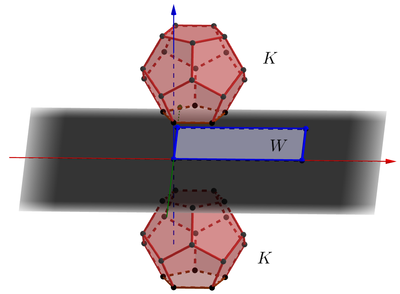# Throwing a Sofa Through the Window

## AbstractA convex polytope K in an initial configuration above thexy-plane; the same polytope in a target configurationbelow the the xy-plane; a window W on the xy-plane.

We study several variants of the problem of moving a convex polytope in three dimensions through a rectangular (and sometimes more general) window. Specifically, we study variants in which the motion is restricted to translations only, discuss situations in which such a motion can be reduced to sliding (translation in a fixed direction) and present efficient algorithms for those variants. We then discuss the case of a window that is unbounded (has two infinite edges) and show that in this case, rotations are not necessary for passing the polytope through the window, an observation that leads to an efficient algorithm for this variant too. Then we study the importance of rotations by an example of a polytope that cannot pass through a certain window by translations only, but it can do so when rotations are allowed. We study also more general convex windows, and obtain some special properties of polytopes that can pass such a convex window. We then study the case of a circular window, and show that, for the regular tetrahedron K, there are two thresholds 1>d1>d2 such that (i) K can slide through the window W if its diameter d is >=1, (ii) K cannot slide through W but can pass through it by a purely translational motion when d1<=d<1, (iii) K cannot pass through W by a purely translational motion but can do it when rotations are allowed when d2<=d<d1, and (iv) K cannot pass through W at all when d<d2. This divides this motion planning problem into three sub-classes, with different capabilities: one dimensional translation (sliding), purely translational motion, and unrestricted motion (with all six degrees of freedom).

• Itay Yehuda
M.Sc. thesis [pdf]

Itay Yehuda
Dan Halperin
Micha Sharir